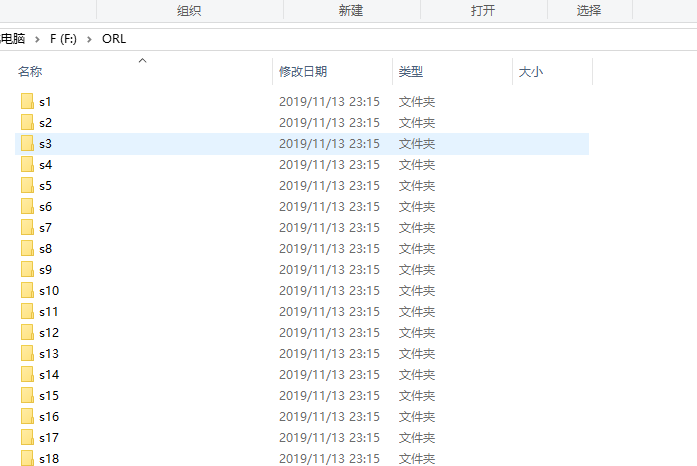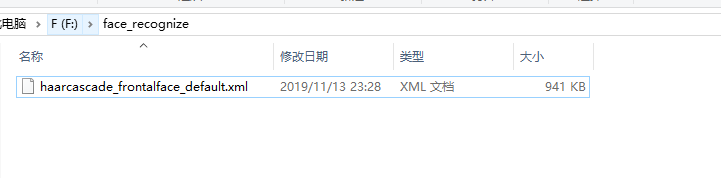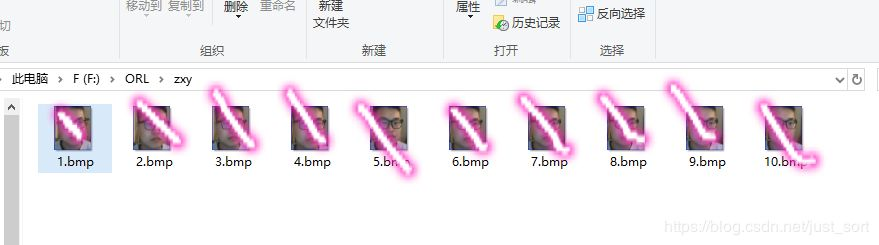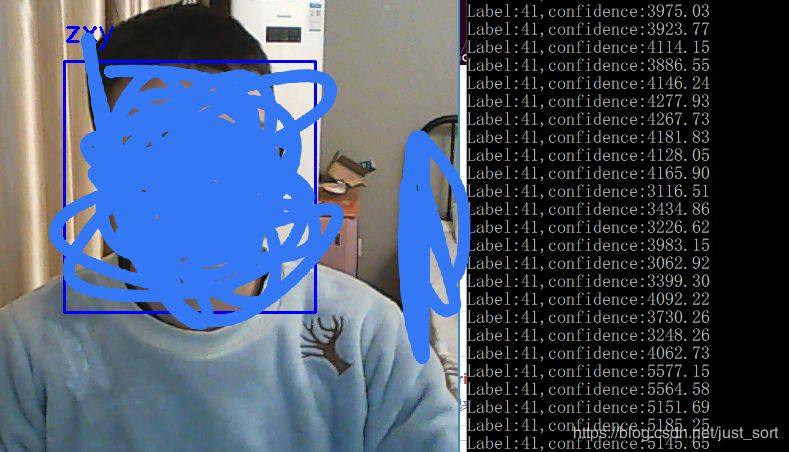# 制作数据集¶#coding=utf-8
# shutil的解释： os模块不仅提供了新建文件、删除文件、查看文件属性的操作功能，
# 还提供了对文件路径的操作功能。但是，对于移动、复制、打包、压缩、解压文件
# 及文件夹等操作，os模块没有提供相关的函数，此时需要用到shutil模块。shutil
# 模块是对os模块中文件操作的补充，是Python自带的关于文件、文件夹、压缩文件
# 的高层次的操作工具，类似于高级API。

import numpy as np
import cv2
import shutil
import os

# 生成自己的人脸数据
def generator(data):
name = input('Input Name: ')
# 如果路径存在则删除
path = os.path.join(data, name)
if os.path.isdir(path):
shutil.rmtree(path) #递归删除文件夹
# 创建文件
os.mkdir(path)
# 创建一个级联分类器，加载一个xml分类器文件，它既可以是Harr特征也可以是LBP特征的分类器
# 打开摄像头
camera = cv2.VideoCapture(1)
cv2.namedWindow('Face')
# 计数
count = 1
while True:
# 读取一帧图像
# 判断图片是否读取成功
if ret:
gray_img = cv2.cvtColor(frame, cv2.COLOR_BGR2GRAY)
#人脸检测
for (x,y,w,h) in faces:
# 在原图像上绘制矩形
cv2.rectangle(frame,(x,y),(x+w,y+h),(255,0,0),2)
# 调整图像大小 和ORL人脸库图像一样大小
f = cv2.resize(frame[y:y+h,x:x+w],(92,112))
# 保存人脸
cv2.imwrite('%s/%s.bmp'%(path,str(count)),f)
count += 1
cv2.imshow('Face', frame)
#如果按下q键则退出
if cv2.waitKey(100) & 0xff == ord('q') :
break
camera.release()
cv2.destroyAllWindows()

if __name__=='__main__':
data = 'F:\\ORL'
generator(data)# 人脸识别¶

OpenCV有3种人脸识别算法，Eigenfaces,Fisherfaces和Local Binary Pattern Histogram。这几个算法都需要对图像或视频中检测到的人脸进行分析，并在识别到人脸的情况下给出人脸类别的概率。我们在实际应用中可以通过卡阈值来完成最后的识别工作。这篇文章主要是介绍特征脸法，特征脸法，本质上其实就是PCA降维，这种算法的基本思路是，把二维的图像先灰度化，转化为一通道的图像，之后再把它首尾相接转化为一个列向量，假设图像大小是20*20的，那么这个向量就是400维，理论上讲组织成一个向量，就可以应用任何机器学习算法了，但是维度太高算法复杂度也会随之升高，所以需要使用PCA算法降维，然后使用简单排序或者KNN都可以。

## 准备数据¶

# 读取
# data表示训练数据集所在的目录，要求图片尺寸一致
# images：[m, height, width] 其中m代表样本个数，height代表图片高度，width代表宽度
# names: 名字的集合
# labels: 标签

images = []
labels = []
names = []

label = 0
#过滤所有的文件夹
for subDirname in os.listdir(data):
subjectPath = os.path.join(data,subDirname)
if os.path.isdir(subjectPath):
#每一个文件夹下存放着一个人的照片
names.append(subDirname)
for fileName in os.listdir(subjectPath):
imgPath = os.path.join(subjectPath,fileName)
images.append(img)
labels.append(label)
label += 1
images = np.asarray(images)
labels = np.asarray(labels)
return images,labels,names


## 构建人脸识别模型¶

def FaceRecognize():
#加载训练数据

model = cv2.face.EigenFaceRecognizer_create()
model.train(X,y)

#打开摄像头
camera = cv2.VideoCapture(1)
cv2.namedWindow('Face')

while(True):
# 读取一帧图像
#判断图片读取成功
if ret:
gray_img = cv2.cvtColor(frame,cv2.COLOR_BGR2GRAY)
#人脸检测

for (x,y,w,h) in faces:
#在原图像上绘制矩形
frame = cv2.rectangle(frame,(x,y),(x+w,y+h),(255,0,0),2)
roi_gray = gray_img[y:y+h,x:x+w]
try:
#宽92 高112
roi_gray = cv2.resize(roi_gray,(92,112),interpolation=cv2.INTER_LINEAR)
params = model.predict(roi_gray)
print('Label:%s,confidence:%.2f'%(params,params))
cv2.putText(frame,names[params],(x,y-20),cv2.FONT_HERSHEY_SIMPLEX,1,255,2)
except:
continue

cv2.imshow('Face',frame)
#如果按下q键则退出
if cv2.waitKey(100) & 0xff == ord('q') :
break
camera.release()
cv2.destroyAllWindows()


pip3 install opencv-contrib-python


# 结果¶# 特征脸法原理¶

• 对图片进行预处理：将图片灰度化，调整到统一尺寸，进行光照归一化等。
• 将图片转换为一个向量：经过灰度化处理的图片是一个矩阵，将这个矩阵中的每一行连到一起，则可以变为一个向量，将该向量转换为列向量。
• 将数据集种的所有图片都转换为向量后，这些数据可以组成一个矩阵，在此基础上进行零均值化处理，就是将所有人脸在对应的维度求平均，得到一个平均脸(average face)向量$\Phi$，每一个人脸向量减去该向量，从而完成零均值化处理。
• 将经过零均值化处理的图像向量组合在一起，可以得到一个矩阵。通过该矩阵可以得到PCA算法中的协方差矩阵。
• 计算协方差矩阵的特征值和特征向量，每一个特征向量的维度与原始图像向量的维度是一致的，因此这些特征向量可以看成是一致的，因此这些特征向量就是所谓的特征脸。

$C=XX^T$

$C'=X^TX$

#encoding=utf-8
import numpy as np
import cv2
import os

class EigenFace(object):
def __init__(self,threshold,dimNum,dsize):
self.threshold = threshold # 阈值暂未使用
self.dimNum = dimNum
self.dsize = dsize

'''
载入图像，灰度化处理，统一尺寸，直方图均衡化
:param fileName: 图像文件名
:param dsize: 统一尺寸大小。元组形式
:return: 图像矩阵
'''
retImg = cv2.resize(img,dsize)
retImg = cv2.cvtColor(retImg,cv2.COLOR_RGB2GRAY)
retImg = cv2.equalizeHist(retImg)
# cv2.imshow('img',retImg)
# cv2.waitKey()
return retImg

def createImgMat(self,dirName):
'''
生成图像样本矩阵，组织形式为行为属性，列为样本
:param dirName: 包含训练数据集的图像文件夹路径
:return: 样本矩阵，标签矩阵
'''
dataMat = np.zeros((10,1))
label = []
for parent,dirnames,filenames in os.walk(dirName):
# print parent
# print dirnames
# print filenames
index = 0
for dirname in dirnames:
for subParent,subDirName,subFilenames in os.walk(parent+'/'+dirname):
for filename in subFilenames:
tempImg = np.reshape(img,(-1,1))
if index == 0 :
dataMat = tempImg
else:
dataMat = np.column_stack((dataMat,tempImg))
label.append(subParent+'/'+filename)
index += 1
return dataMat,label

def PCA(self,dataMat,dimNum):
'''
PCA函数，用于数据降维
:param dataMat: 样本矩阵
:param dimNum: 降维后的目标维度
:return: 降维后的样本矩阵和变换矩阵
'''
# 均值化矩阵
meanMat = np.mat(np.mean(dataMat,1)).T
print '平均值矩阵维度',meanMat.shape
diffMat = dataMat-meanMat
# 求协方差矩阵，由于样本维度远远大于样本数目，所以不直接求协方差矩阵，采用下面的方法
covMat = (diffMat.T*diffMat)/float(diffMat.shape) # 归一化
#covMat2 = np.cov(dataMat,bias=True)
#print '基本方法计算协方差矩阵为',covMat2
print '协方差矩阵维度',covMat.shape
eigVals, eigVects = np.linalg.eig(np.mat(covMat))
print '特征向量维度',eigVects.shape
print '特征值',eigVals
eigVects = diffMat*eigVects
eigValInd = np.argsort(eigVals)
eigValInd = eigValInd[::-1]
eigValInd = eigValInd[:dimNum] # 取出指定个数的前n大的特征值
print '选取的特征值',eigValInd
eigVects = eigVects/np.linalg.norm(eigVects,axis=0) #归一化特征向量
redEigVects = eigVects[:,eigValInd]
print '选取的特征向量',redEigVects.shape
print '均值矩阵维度',diffMat.shape
lowMat = redEigVects.T*diffMat
print '低维矩阵维度',lowMat.shape
return lowMat,redEigVects

def compare(self,dataMat,testImg,label):
'''
比较函数，这里只是用了最简单的欧氏距离比较，还可以使用KNN等方法，如需修改修改此处即可
:param dataMat: 样本矩阵
:param testImg: 测试图像矩阵，最原始形式
:param label: 标签矩阵
:return: 与测试图片最相近的图像文件名
'''
testImg = cv2.resize(testImg,self.dsize)
testImg = cv2.cvtColor(testImg,cv2.COLOR_RGB2GRAY)
testImg = np.reshape(testImg,(-1,1))
lowMat,redVects = self.PCA(dataMat,self.dimNum)
testImg = redVects.T*testImg
print '检测样本变换后的维度',testImg.shape
disList = []
testVec = np.reshape(testImg,(1,-1))
for sample in lowMat.T:
disList.append(np.linalg.norm(testVec-sample))
print disList
sortIndex = np.argsort(disList)
return label[sortIndex]

def predict(self,dirName,testFileName):
'''
预测函数
:param dirName: 包含训练数据集的文件夹路径
:param testFileName: 测试图像文件名
:return: 预测结果
'''